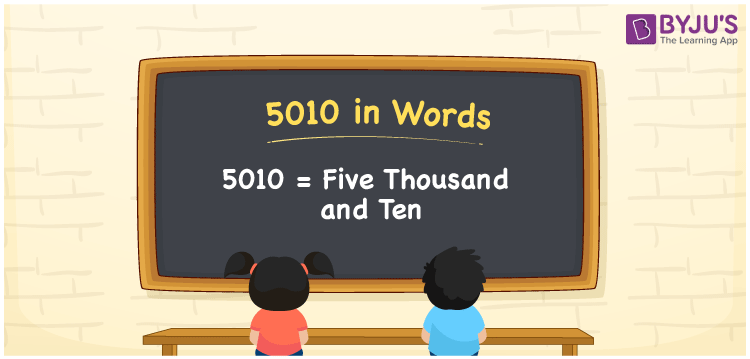# 5010 in words

5010 in words is written as Five Thousand and Ten. 5010 represents the count or value. The article on Counting Numbers can give you an idea about count or counting. The number 5010 is used in expressions that relate to money, distance, length and others. Let us consider an example for 5010. ”I had to delete Five Thousand and Ten spam mails”.

 5010 in words Five Thousand and Ten Five Thousand and Ten in Numbers 5010

## 5010 in English Words## How to Write 5010 in Words?

We can convert 5010 to words using a place value chart. The number 5010 has 4 digits, so let’s make a chart that shows the place value up to 4 digits.

 Thousands Hundreds Tens Ones 5 0 1 0

Thus, we can write the expanded form as:

5 × Thousand + 0 × Hundred + 1 × Ten + 0 × One

= 5 × 1000 + 0 × 100 + 1 × 10 + 0 × 1

= 5010

= Five Thousand and Ten.

5010 is the natural number that is succeeded by 5009 and preceded by 5011.

5010 in words – Five Thousand and Ten.

Is 5010 an odd number? – No.

Is 5010 an even number? – Yes.

Is 5010 a perfect square number? – No.

Is 5010 a perfect cube number? – No.

Is 5010 a prime number? – No.

Is 5010 a composite number? – Yes.

## Solved Example

1. Write the number 5010 in expanded form

Solution: 5 × 1000 + 0 × 100 + 1 × 10 + 0 × 1

We can write 5010 = 5000 +0300 + 10 + 0

= 5 × 1000 + 0 × 100 + 1 × 10 + 0 × 1

## Frequently Asked Questions on 5010 in words

Q1

### How to write 5010 in words?

5010 in words is written as Five Thousand and Ten.
Q2

### Is 5010 a perfect square number?

No. 5010 is not a perfect square number.
Q3

### Is 5010 a prime number?

No. 5010 is not a prime number.